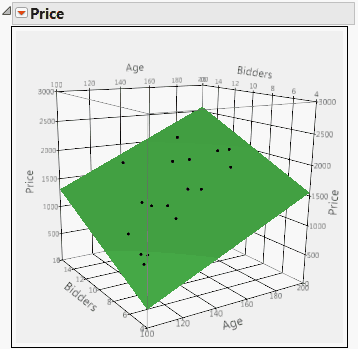Publication date: 04/12/2021

## Multiple Linear Regression

Effects to be entered: Selected columns

1. In the Select Columns list, select the continuous effects of interest.

2. Click Add.

Figure 2.7 shows a surface profiler plot of the data and of the multiple linear regression fit to the data for the Grandfather Clocks.jmp sample data table. The model effects are Age and Bidders. The response is Price. You can obtain the plot by running the data table script Fit Model with Surface Profiler Plot.

Figure 2.7 Model Fit for a Multiple Linear Regression Model with Two Predictors#### Example of Multiple Linear Regression Model

See also Example of a Regression Analysis Using Fit Model for an example with several predictors.

Want more information? Have questions? Get answers in the JMP User Community (community.jmp.com).